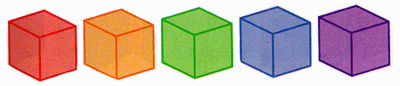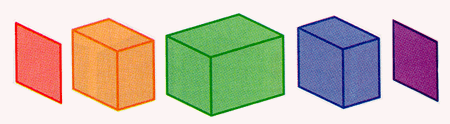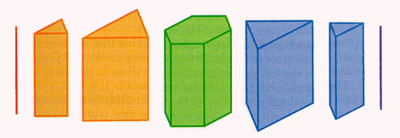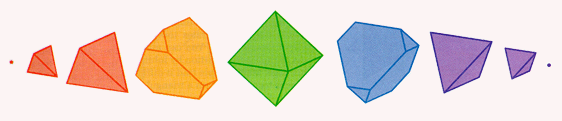## Slicing the Hypercube

We have already seen how a hypercube would appear if it penetrated our universe cube first: at the first instant, we would see an ordinary cube with 8 bright vertices, then a dull cube for a while, and finally another cube with 8 bright vertices. What would happen if the hypercube came through our universe from another direction, for example square first or edge first or corner first? For such questions, the modern graphics computer is ideally suited. We can exhibit the slices even though we cannot construct the objects themselves that are being sliced!

We can imagine four-dimensional versions of Froebel's geometrical gifts, a hyperball and a hypercube suspended "above" our three-dimensional world, thought of as the analogue of the surface of the water. How will the appearance of the slices change as the water level rises to cover the objects?

The slicing sequence of the hyperball has already been compared to the inflation and deflation of a spherical balloon. No matter which way the hyperball is rotated, we will get the same sequence. This is analogous to the appearance of the slices of an ordinary ball passing through Flatland. But just as the slices of an ordinary cube depend on the orientation of the cube with respect to the slicing plane, so too do the slices of the hypercube.

If a cube comes through the horizontal plane square first, we see a square for a while, and if the hypercube comes through our space cube first, we see a cube for a while.Slices of the hypercube starting with a cube.

A cube coming through edge first was sliced into a series of rectangles. Thinking about the formation of the rectangular slices will guide us to the slices of a hypercube. The squares perpendicular to the leading edge are sliced by a line parallel to one of their diagonals. The slices of each of these squares are line segments: the segments start at a point, grow to a diagonal of the square, and shrink back to a point. Thus the rectangular slices of the cube will have one set of unchanging edges equal in length to an edge of the cube, and another set growing from a point to the length of a diagonal and shrinking back to zero. The 8 vertices of the cube are partitioned into three sets: 2 in the beginning edge, 4 in the largest rectangular slice, and 2 in the final edge.

The comparable slicing sequence for the hypercube starts with a square face, which expands in a series of rectangular prisms all having square bases of the same size. The rectangular sides grow until they reach the length of a diagonal of the original square and then shrink back down to zero. The 16 vertices of the hypercube are thus arranged in three sets: 4 at the beginning, then 8 in the middle, and 4 at the end.Slices of the hypercube starting with a square.

Slicing an ordinary cube corner first yields a point expanding to a triangle, changing to a hexagon, reverting back to a triangle, and shrinking down to a point. The 8 vertices of the cube are arranged in four sets of, in order, 1, 3, 3, and 1 vertices. If we slice a hypercube edge first, we see an edge expanding to a triangular prism with height equal to the length of the original edge. The triangular prism changes to a hexagonal prism, reverts back to a triangular prism, and shrinks down to an edge. The 16 vertices of the hypercube are arranged in four sets of, in order, 2, 6, 6, and 2 vertices.Slices of the hypercube starting with an edge.

Finally, if a hypercube comes through vertex first, we start with a single vertex, which expands to form a small triangular pyramid. This tetrahedron expands until it reaches a level containing 4 vertices of the hypercube. Then it experiences truncation as the corners of the tetrahedron are cut off, and three-eighths of the way through, the slice is a solid figure having four equilateral triangles and four regular hexagons as faces. Each hexagon is the slice exactly halfway through one of the cubes in the boundary of the hypercube. This solid is a semi-regular polyhedron known to Archimedes as far back as the third century B.C.Slices of the hypercube starting with a vertex.

As the slicing plane continues to the position exactly halfway through the hypercube, the four hexagons become triangles, and, together with the previous four triangles, they form a perfect regular octahedron, a Platonic solid that has as its vertices 6 of the vertices of the original hypercube. The second half of the sequence of slices is a reversal of the first half: the original four triangles become hexagons, and the hexagons shrink as the triangles expand until finally there forms a tetrahedron, which shrinks down to the final point of the hypercube. In this final slicing sequence, the 16 vertices of the hypercube are arranged in five different levels: first 1 vertex, then 4 (for a tetrahedron), then 6 (for the octahedron), then 4, and then finally 1.

For a square going through Lineland corner first, the sequence of groups of vertices is 1, 2, 1; for a cube going corner first through Flatland, the vertices appear in successive slices in groups of 1, 3, 3, 1. The corresponding hypercube sequence is 1, 4, 6, 4, 1. This sequence has already appeared as the coefficients in the binomial theorem, and it will arise again when we deal with the coordinates for the hypercube in Chapter 8.

Already in the last century, mathematicians had fashioned models to show the sequence of hypercube slices in various directions. Mrs. Alicia Boole Stott was a genius at predicting the slicing sequences of four-dimensional polyhedra, even though she had no formal training in the techniques of higher-dimensional geometry. In our day we finally have a chance to investigate the objects that our ancestors could only dream about and represent in lifeless models. Interactive computer graphics puts us into direct visual contact with slices of four-dimensional cubes. It is up to us to learn how to interpret these images, and to try to overcome the limitation of our own three-dimensional perspective.